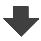Search:

# Acid-base theory

## pH and pOH

### Exercise 1

In the following multiple-choice questionnaire, $H_3O^+$ is often abbreviated by $H^+$ ( which is quite wrong, because $H^+$ is always hydrated in the water!)

Which of the following species is amphoteric ? i) $H^+$ ii) $OH^-$ iii) $H_2O$ iv) $H_3O^+ a) iii) alone b) ii) and iii) alone c) iii) and iv) alone d) ii), iii) and iv) alone e) all a) is correct ,$OH^-$is not amphoteric, because it has no acid caracter (too big a$pKa\$)